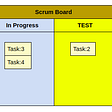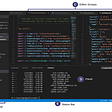# Coding Algorithms — QuickSelect

In many interviews, interviewers likes to test a candidates space complexity skills apart from time complexity. Although time complexity optimization remains the most sought after problem solving goal, but space complexity optimization can be critical in real life programming especially when working with large datasets in memory.

In this post we look at some problems that leverages the quickselect technique to modify arrays or strings in place or as we like to say in O(1) space complexity.

In the quickselect technique, an element is placed at its correct position in an array, by swapping it with the first occurrence of an element not in its correct position.

Problem 1:

Given an array of integers and an integer X. Modify the array in-place so that all integers less than X comes before X and integers greater than X comes after X in the final array.

`Input: arr=[4,1,9,0,6,5,4,3,1,2,11,8,8], X=7Output: [4,1,0,6,5,4,3,1,2,9,11,8,8]`

Note that the ordering among the smaller and greater elements do not matter for this problem.

Solution:

Maintain a variable V to track the index of the first element greater than X.

If we see an element which is smaller than equal to X and the variable V is a valid index i.e. we have seen at-least one greater element then swap the current element with index V.

After swap, increment index V by 1.

Because if the current index is I, then V < I and all indices between V and I have elements greater than arr[I]. Assuming that if there was an index J s.t. V < J < I and arr[J] < arr[I], then we would have already swapped J with V, in which case V would not be the first index greater than X.

Time complexity is O(N). Space complexity is O(1).

Problem 2:

Given an array `nums` with `n` objects colored red, white, or blue, sort them in-place so that objects of the same color are adjacent, with the colors in the order red, white, and blue.

We will use the integers `0`, `1`, and `2` to represent the color red, white, and blue, respectively.

You must solve this problem without using the library’s sort function.

Example 1:

`Input: nums = [2,0,2,1,1,0]Output: [0,0,1,1,2,2]`

Solution:

This is similar to the 1st example, only difference is that we need to maintain 2 variables ‘one_first_index’ and ‘two_first_index’ because we need to sort the array as well.

For the current index I, if it is 1 and two_first_index < I, then we need to swap I with two_first_index and increment two_first_index by 1.

Similarly if at current index I, element is 0 and if one_first_index < I, then there could be a valid two_first_index, s.t. one_first_index < two_first_index < I (because for all indices before I it has already been sorted). Put 0 at one_first_index, 1 at two_first_index and 2 at I and increment one_first_index as well as two_first_index by 1.

Else if either of one_first_index < I or two_first_index < I, then swap and correspondingly increment the index by 1.

Time complexity is O(N). Space complexity is O(1).

Problem 3:

Given a string S containing additional spaces. Remove the extra spaces in-place without allocating any additional string or data structure.

`Input:  "   This    is an      example   text "Output: "This is an example text"`

Solution:

Since string is immutable in python, we will convert the string to an array of characters and update the array in-place.

The idea is similar to above.

Maintain a variable to track the first index of space in the string. But need to take care of the scenarios where the first space is at the beginning of string vs. first space is after first word.

Also each word must be followed by only one space. Count the number of consecutive spaces. If there are more than 1 space in sequence, then skip those extra spaces else swap the character at index I with index ‘first_space’.

Time complexity is O(N). Space complexity is O(1).

Problem 4:

Given a sorted array of integers containing duplicates. Remove the duplicates and modify the array in-place.

`Input: [1,1,1,1,2,2,2,5,5,6]Output: [1,2,5,6]`

Solution:

Maintain an index ‘dup_index’ which is the first index of duplicate element.

Time complexity is O(N). Space complexity is O(1).

Problem 5:

Given an array of characters `chars`, compress it using the following algorithm:

Begin with an empty string `s`. For each group of consecutive repeating characters in `chars`:

• If the group’s length is `1`, append the character to `s`.
• Otherwise, append the character followed by the group’s length.

The compressed string `s` should not be returned separately, but instead, be stored in the input character array `chars`. Note that group lengths that are `10` or longer will be split into multiple characters in `chars`.

After you are done modifying the input array, return the new length of the array.

You must write an algorithm that uses only constant extra space.

Example 1:

`Input: chars = ["a","a","b","b","c","c","c"]Output: Return 6, and the first 6 characters of the input array should be: ["a","2","b","2","c","3"]Explanation: The groups are "aa", "bb", and "ccc". This compresses to "a2b2c3".`

Solution:

Approach is similar to above problem but instead of discarding the counts of duplicates, replace sufficient duplicates by the count and increment ‘dup_index’ everytime a duplicate is replaced by a character of count.

--

--

--

## More from Abhijit Mondal

Engineer

Love podcasts or audiobooks? Learn on the go with our new app.

## Debugging in Production—How to move fast without breaking things## INSIDE HARROW COUNCIL’S PEOPLE-FIRST IT TRANSFORMATION## Setting up for Success in Multi-Team Development## Rust Programing For Beginners in 6 Mins!## Scrum vs Kanban## PHP — P6: Integers## BEM Is Terrible## Visual Studio Code: Show hidden folderEngineer

## Coding Algorithms — Monotonic Stacks and Queues## The Main Search and Sort Algorithms